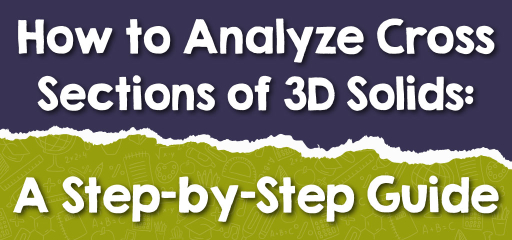# How to Analyze Cross Sections of 3D Solids: A Step-by-Step Guide

Imagine cutting a loaf of bread, revealing a myriad of shapes and patterns with each slice. This process resembles the fascinating world of solids and their cross sections in geometry. By slicing or 'sectioning' these three-dimensional figures, we uncover a range of two-dimensional shapes that shed light on the structure and properties of the original solid. This guide aims to pull back the curtain on this captivating topic, diving into the heart of various solids and unveiling the surprises they house within. So, grab your geometric 'knife' and let's slice through the world of 3D shapes!## Step-by-step Guide: Solids and Their Cross Sections

Rectangular Prism: When cut parallel to its base, a rectangular prism will yield rectangles. If cut perpendicular to one of its edges, the section could be a square or rectangle, depending on the orientation.

Cylinder: A horizontal cut will always yield a circle. Vertical cuts, depending on orientation, can result in rectangles or ellipses.

Sphere: Any planar cut through the center of a sphere will always produce a circle.

Cube: For a cube, any cut that’s parallel to a face will reveal a square. Non-parallel cuts can result in rectangles, parallelograms, or even triangles, depending on the angle and orientation of the cut.

Pyramid: With a pyramid, horizontal cuts will produce polygons that resemble the base but are smaller in size. A vertical section through the apex will result in a triangle.

Cone: For a cone, a horizontal cut will always produce a circle. A vertical cut, however, that goes through the apex will reveal a sector of a circle, which appears as a curved triangle.

### Examples

Example 1:
If a cylinder with a base diameter of $$8 \text{ cm}$$ is sliced vertically through its center, what shape is revealed?

Solution:
The cross section will be a rectangle with a height equal to the cylinder’s height and a width of $$8 \text{ cm}$$ (the diameter).

Example 2:
If a rectangular prism with dimensions $$10 \text{ cm} \times 5 \text{ cm} \times 4 \text{ cm}$$ is sliced parallel to its longest side, what shape is revealed?

Solution:
The cross section will be a rectangle measuring $$5 \text{ cm} \times 4 \text{ cm}$$.

Example 3:
If a sphere with a radius of $$7 \text{ cm}$$ is sectioned through its center, what is the diameter of the resulting circle?

Solution:
The diameter is $$2 \times 7 \text{ cm} = 14 \text{ cm}$$.

Example 4:
If you slice a cube diagonally from one corner to the opposite corner on the same face, what shape will you get?

Solution:
The cross section will be a triangle.

Example 5:
If a square-based pyramid is sectioned parallel to its base about midway up, what is the resulting cross section?

Solution:
The cross section will be a square, but smaller than the base of the pyramid.

Example 6:
If a cone is sliced vertically, passing through its apex and base, what shape is revealed?

Solution:
The cross section will be a sector of a circle, also described as a ‘curved triangle’.

### Practice Questions:

1. What will be the shape of a cross section if a cone is sliced horizontally near its base?
2. If a pyramid with a square base is sectioned parallel to its base halfway up, what shape will the cross section reveal?
3. What is the shape of the cross section when a cylinder is cut at an angle, not parallel to its base or directly vertical?
4. If a cube has an edge length of $$6 \text{ cm}$$ and is sliced parallel to one of its faces, but only $$2 \text{ cm}$$ from the face, what is the area of the resulting cross section?
5. What will be the shape of a cross section if a triangular pyramid is sliced horizontally near its apex?
6. When a cone is cut horizontally close to its tip, what shape does the cross section reveal, and how does it relate to the base of the cone?

1. A smaller circle.
2. A smaller square.
3. An ellipse.
4. $$36 \text{ cm}^2$$ (It’s a square with the same edge length as the cube).
5. A smaller triangle.
6. A circle, much smaller than the base.

### What people say about "How to Analyze Cross Sections of 3D Solids: A Step-by-Step Guide - Effortless Math: We Help Students Learn to LOVE Mathematics"?

No one replied yet.

X
30% OFF

Limited time only!

Save Over 30%

SAVE $5 It was$16.99 now it is \$11.99